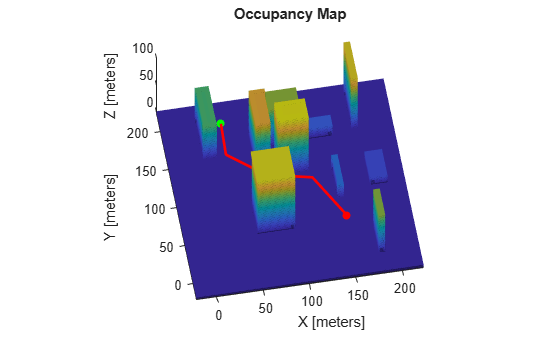# plan

Plan path between two states

Since R2019b

## Syntax

``path = plan(planner,startState,goalState)``
``[path,solutionInfo] = plan(planner,startState,goalState)``

## Description

````path = plan(planner,startState,goalState)` returns a `path` from the start state to the goal state.```

example

````[path,solutionInfo] = plan(planner,startState,goalState)` also returns `solutionInfo` that contains the solution information of the path planning.```

## Examples

collapse all

Create a state space.

`ss = stateSpaceSE2;`

Create an `occupancyMap`-based state validator using the created state space.

`sv = validatorOccupancyMap(ss);`

Create an occupancy map from an example map and set map resolution as 10 cells/meter.

```load exampleMaps map = occupancyMap(simpleMap,10); sv.Map = map;```

Set validation distance for the validator.

`sv.ValidationDistance = 0.01;`

Update state space bounds to be the same as map limits.

`ss.StateBounds = [map.XWorldLimits;map.YWorldLimits;[-pi pi]];`

Create the path planner and increase the maximum connection distance.

`planner = plannerRRT(ss,sv,MaxConnectionDistance=0.3);`

Set the start and goal states.

```start = [0.5 0.5 0]; goal = [2.5 0.2 0];```

Plan a path with default settings.

```rng(100,'twister'); % for repeatable result [pthObj,solnInfo] = plan(planner,start,goal);```

Visualize the results.

```show(map) hold on % Tree expansion plot(solnInfo.TreeData(:,1),solnInfo.TreeData(:,2),'.-') % Draw path plot(pthObj.States(:,1),pthObj.States(:,2),'r-','LineWidth',2)```Load a 3-D occupancy map of a city block into the workspace. Specify the threshold to consider cells as obstacle-free.

```mapData = load("dMapCityBlock.mat"); omap = mapData.omap; omap.FreeThreshold = 0.5;```

Inflate the occupancy map to add a buffer zone for safe operation around the obstacles.

`inflate(omap,1)`

Create an SE(3) state space object with bounds for state variables.

`ss = stateSpaceSE3([0 220;0 220;0 100;inf inf;inf inf;inf inf;inf inf]);`

Create a 3-D occupancy map state validator using the created state space. Assign the occupancy map to the state validator object. Specify the sampling distance interval.

```sv = validatorOccupancyMap3D(ss, ... Map = omap, ... ValidationDistance = 0.1);```

Create a RRT path planner with increased maximum connection distance and reduced maximum number of iterations. Specify a custom goal function that determines that a path reaches the goal if the Euclidean distance to the target is below a threshold of 1 meter.

```planner = plannerRRT(ss,sv, ... MaxConnectionDistance = 50, ... MaxIterations = 1000, ... GoalReachedFcn = @(~,s,g)(norm(s(1:3)-g(1:3))<1), ... GoalBias = 0.1);```

Specify start and goal poses.

```start = [40 180 25 0.7 0.2 0 0.1]; goal = [150 33 35 0.3 0 0.1 0.6];```

Configure the random number generator for repeatable result.

`rng(1,"twister");`

Plan the path.

`[pthObj,solnInfo] = plan(planner,start,goal);`

Visualize the planned path.

```show(omap) axis equal view([-10 55]) hold on % Start state scatter3(start(1,1),start(1,2),start(1,3),"g","filled") % Goal state scatter3(goal(1,1),goal(1,2),goal(1,3),"r","filled") % Path plot3(pthObj.States(:,1),pthObj.States(:,2),pthObj.States(:,3), ... "r-",LineWidth=2)```## Input Arguments

collapse all

Path planner, specified as a `plannerRRT` object or a `plannerRRTStar` object.

Start state of the path, specified as an N-element real-valued vector. N is the dimension of the state space.

Example: `[1 1 pi/6]`

Example: `[40 180 25 0.7 0.2 0 0.1]`

Data Types: `single` | `double`

Goal state of the path, specified as an N-element real-valued vector. N is the dimension of the state space.

Example: `[2 2 pi/3]`

Example: `[150 33 35 0.3 0 0.1 0.6]`

Data Types: `single` | `double`

## Output Arguments

collapse all

An object that holds the planned path information, returned as a `navPath` object.

Solution Information, returned as a structure. The fields of the structure are:

Fields of `solutionInfo`

FieldsDescription
`IsPathFound`Indicates whether a path is found. It returns as `1` if a path is found. Otherwise, it returns `0`.
`ExitFlag`

Indicates the terminate status of the planner, returned as

• 1 — if the goal is reached

• 2 — if the maximum number of iterations is reached

• 3 — if the maximum number of nodes is reached

`NumNodes`Number of nodes in the search tree when the planner terminates (excluding the root node).
`NumIterations`Number of "extend" routines executed.
`TreeData`A collection of explored states that reflects the status of the search tree when planner terminates. Note that `NaN` values are inserted as delimiters to separate each individual edge.
`PathCosts`

Contains the cost of the path at each iteration. Value for iterations when path has not reached the goal is denoted by a `NaN`. Size of the array is `NumIterations`-by-1. Last element contains the cost of the final path.

Note

This field is applicable only for `plannerRRTStar` object.

Data Types: `structure`

## Version History

Introduced in R2019b